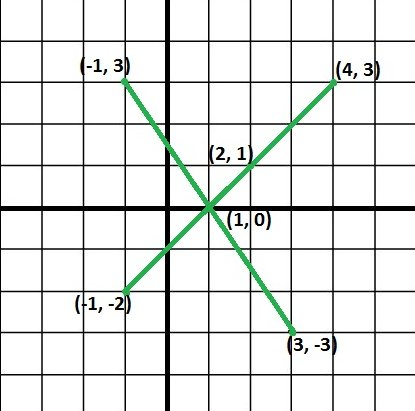# Minimum lines to cover all points

Given N points in 2-dimensional space, we need to print the count of the minimum number of lines which traverse through all these N points and which go through a specific (xO, yO) point also.

Examples:

```If given points are (-1, 3), (4, 3), (2, 1), (-1, -2),
(3, -3) and (xO, yO) point is (1, 0) i.e. every line
must go through this point.
Then we have to draw at least two lines to cover all
these points going through (xO, yO) as shown in below
diagram.```

## Recommended: Please try your approach on {IDE} first, before moving on to the solution.

We can solve this problem by considering the slope of all points with (xO, yO). If two distinct points have the same slope with (xO, yO) then they can be covered with same line only so we can track slope of each point and whenever we get a new slope we will increase our line count by one.
In below code slope is stored as a pair of integer to get rid of the precision problem and a set is used to keep track of occurred slopes.
Please see below code for better understanding.

## CPP

 `// C++ program to get minimum lines to cover ` `// all the points ` `#include ` `using` `namespace` `std; ` ` `  `//    Utility method to get gcd of a and b ` `int` `gcd(``int` `a, ``int` `b) ` `{ ` `    ``if` `(b == 0) ` `        ``return` `a; ` `    ``return` `gcd(b, a % b); ` `} ` ` `  `//    method returns reduced form of dy/dx as a pair ` `pair<``int``, ``int``> getReducedForm(``int` `dy, ``int` `dx) ` `{ ` `    ``int` `g = gcd(``abs``(dy), ``abs``(dx)); ` ` `  `    ``//    get sign of result ` `    ``bool` `sign = (dy < 0) ^ (dx < 0); ` ` `  `    ``if` `(sign) ` `        ``return` `make_pair(-``abs``(dy) / g, ``abs``(dx) / g); ` `    ``else` `        ``return` `make_pair(``abs``(dy) / g, ``abs``(dx) / g); ` `} ` ` `  `/*    method returns minimum number of lines to ` `    ``cover all points where all lines goes ` `    ``through (xO, yO) */` `int` `minLinesToCoverPoints(``int` `points[], ``int` `N, ` `                                   ``int` `xO, ``int` `yO) ` `{ ` `    ``//    set to store slope as a pair ` `    ``set< pair<``int``, ``int``> > st; ` `    ``pair<``int``, ``int``> temp; ` `    ``int` `minLines = 0; ` ` `  `    ``//    loop over all points once ` `    ``for` `(``int` `i = 0; i < N; i++) ` `    ``{ ` `        ``//    get x and y co-ordinate of current point ` `        ``int` `curX = points[i]; ` `        ``int` `curY = points[i]; ` ` `  `        ``temp = getReducedForm(curY - yO, curX - xO); ` ` `  `        ``// if this slope is not there in set, ` `        ``// increase ans by 1 and insert in set ` `        ``if` `(st.find(temp) == st.end()) ` `        ``{ ` `            ``st.insert(temp); ` `            ``minLines++; ` `        ``} ` `    ``} ` ` `  `    ``return` `minLines; ` `} ` ` `  `// Driver code to test above methods ` `int` `main() ` `{ ` `    ``int` `xO, yO; ` `    ``xO = 1; ` `    ``yO = 0; ` ` `  `    ``int` `points[] = ` `    ``{ ` `        ``{-1, 3}, ` `        ``{4, 3}, ` `        ``{2, 1}, ` `        ``{-1, -2}, ` `        ``{3, -3} ` `    ``}; ` ` `  `    ``int` `N = ``sizeof``(points) / ``sizeof``(points); ` `    ``cout << minLinesToCoverPoints(points, N, xO, yO); ` `    ``return` `0; ` `} `

## Python3

 `# Python3 program to get minimum lines to cover ` `# all the points ` ` `  `# Utility method to get gcd of a and b ` `def` `gcd(a, b): ` `    ``if` `(b ``=``=` `0``): ` `        ``return` `a ` `    ``return` `gcd(b, a ``%` `b) ` ` `  `# method returns reduced form of dy/dx as a pair ` `def` `getReducedForm(dy, dx): ` `    ``g ``=` `gcd(``abs``(dy), ``abs``(dx)) ` ` `  `    ``# get sign of result ` `    ``sign ``=` `(dy < ``0``) ^ (dx < ``0``) ` ` `  `    ``if` `(sign): ` `        ``return` `(``-``abs``(dy) ``/``/` `g, ``abs``(dx) ``/``/` `g) ` `    ``else``: ` `        ``return` `(``abs``(dy) ``/``/` `g, ``abs``(dx) ``/``/` `g) ` ` `  `# /* method returns minimum number of lines to ` `#     cover all points where all lines goes ` `#     through (xO, yO) */ ` `def` `minLinesToCoverPoints(points, N, xO, yO): ` `     `  `    ``# set to store slope as a pair ` `    ``st ``=` `dict``() ` `    ``minLines ``=` `0` ` `  `    ``# loop over all points once ` `    ``for` `i ``in` `range``(N): ` `         `  `        ``# get x and y co-ordinate of current point ` `        ``curX ``=` `points[i][``0``] ` `        ``curY ``=` `points[i][``1``] ` ` `  `        ``temp ``=` `getReducedForm(curY ``-` `yO, curX ``-` `xO) ` ` `  `        ``# if this slope is not there in set, ` `        ``# increase ans by 1 and insert in set ` `        ``if` `(temp ``not` `in` `st): ` `            ``st[temp] ``=` `1` `            ``minLines ``+``=` `1` ` `  `    ``return` `minLines ` ` `  `# Driver code  ` `xO ``=` `1` `yO ``=` `0` ` `  `points ``=``[[``-``1``, ``3``], ` `         ``[``4``, ``3``], ` `         ``[``2``, ``1``], ` `         ``[``-``1``, ``-``2``], ` `         ``[``3``, ``-``3``]] ` ` `  `N ``=` `len``(points) ` `print``(minLinesToCoverPoints(points, N, xO, yO)) ` ` `  `# This code is contributed by mohit kumar 29 `

Output:

```2
```

This article is contributed by Utkarsh Trivedi. If you like GeeksforGeeks and would like to contribute, you can also write an article using contribute.geeksforgeeks.org or mail your article to contribute@geeksforgeeks.org. See your article appearing on the GeeksforGeeks main page and help other Geeks.

Attention reader! Don’t stop learning now. Get hold of all the important DSA concepts with the DSA Self Paced Course at a student-friendly price and become industry ready.

My Personal Notes arrow_drop_up

Improved By : mohit kumar 29

Article Tags :
Practice Tags :

1

Please write to us at contribute@geeksforgeeks.org to report any issue with the above content.